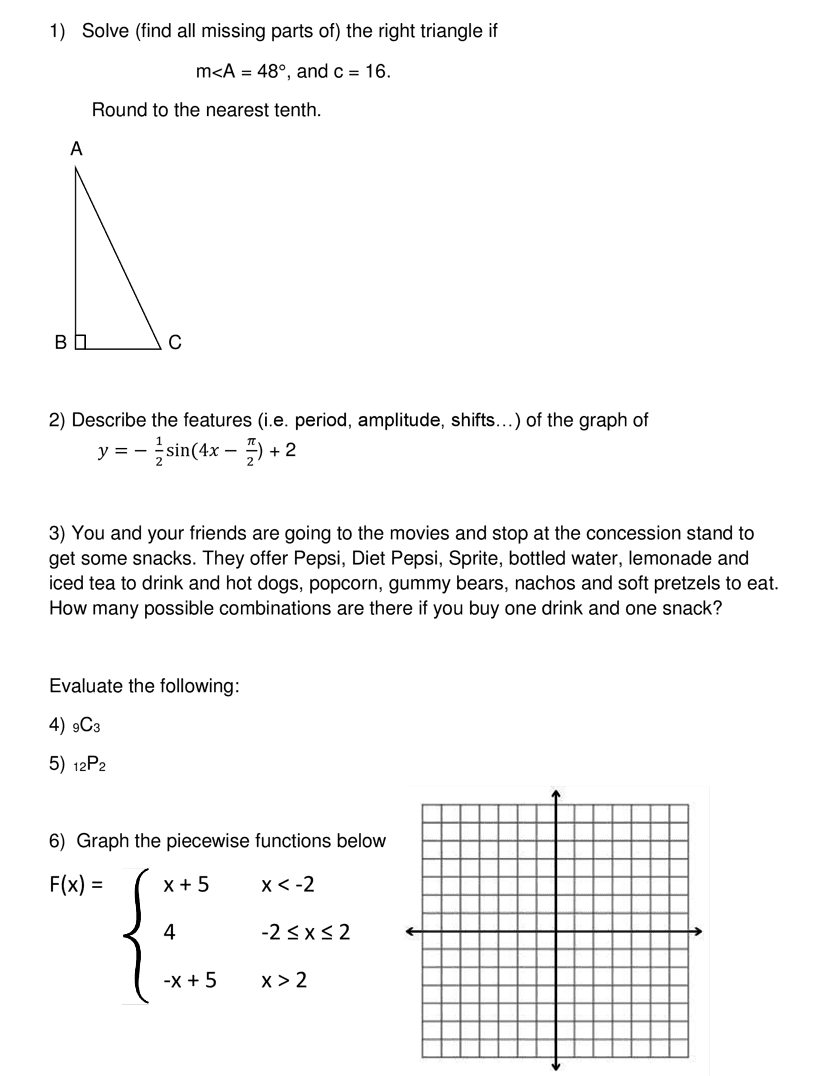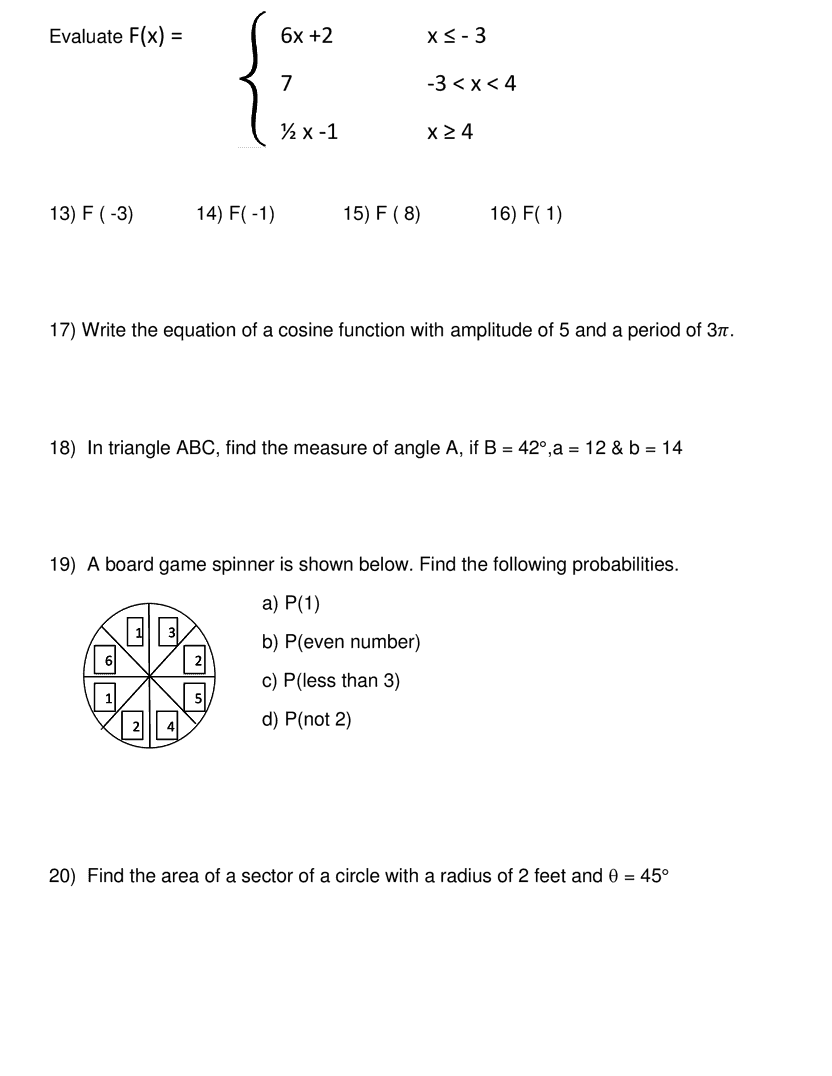Kindergarten
1st
2nd
3rd
4th
5th
6th
7th
8th
9th
10th
11th
12th
Higher Ed
Other
Subjects
ELA
Math
Science
Social Studies
Art
Computer Science
French
German
Music
Physical Education
Spanish
Other
Private Library
AFM Midterm Review
starstarstarstarstarstarstarstarstarstar
by Kristen Lanning
| 32 Questions
Note from the author:
Midterm Review AFM
zoom in1
10 pts
What is the measure of <C?
2
10 pts
What is the measure of side a to two decimal places?
3
10 pts
What is the measure of side b to two decimal places?
4
10 pts
Is the function positive or negative?
5
10 pts
What is the amplitude of the function?
6
10 pts
What is the period of the function? (Use "pi" in your response.)
7
10 pts
**What is the phase shift of the function? Give your answer in the form "value, direction." (Use "pi" in your answer)
8
10 pts
What is the vertical shift of the function? Give your answer in the form value, direction.
9
10 pts
10
10 pts
11
10 pts
12
10 pts
Use the graph and tools below to graph the piecewise functions.
zoom in13
10 pts
Round your answer to two decimal places.
14
10 pts
What is the cosine in reduced fraction form?
15
10 pts
What is the tangent in reduced fraction form?
16
10 pts
Put your answer in the form "\$0.00, yes or no"
17
10 pts
18
10 pts
19
10 pts
Use "pi" in your response.
20
10 pts
Give your response as a reduced fraction.
21
10 pts
Give your answer to two decimal places.
zoom in22
10 pts
23
10 pts
24
10 pts
25
10 pts
26
10 pts
Give your answer in the form y=acos(bx). Use fractions instead of decimals.
27
10 pts
Round to the nearest whole number.
28
10 pts
Give your answer in reduced fraction form.
29
10 pts
Give your answer in reduced fraction form.
30
10 pts
Give your answer in reduced fraction form.
31
10 pts
Give your answer in reduced fraction form.
32
10 pts
Give your answer to two decimal places.
Add to my formatives list